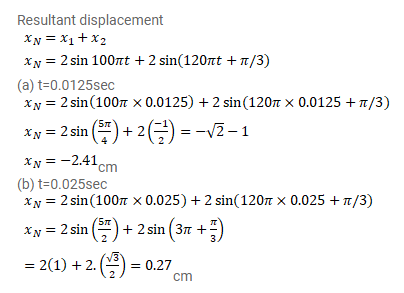# A particle is subjected to two simple harmonic motions given by

Question:

A particle is subjected to two simple harmonic motions given by $x_{1}=2.0 \sin (100 \pi t)$ and $x_{2}=2.0 \sin (120 \pi t+\pi / 3)$

where $x$ is in centimeter and $t$ in second. Find the displacement of the particle at

$(a) t=0.0125,(b) t=0.025$.

Solution: